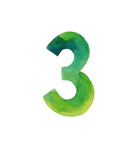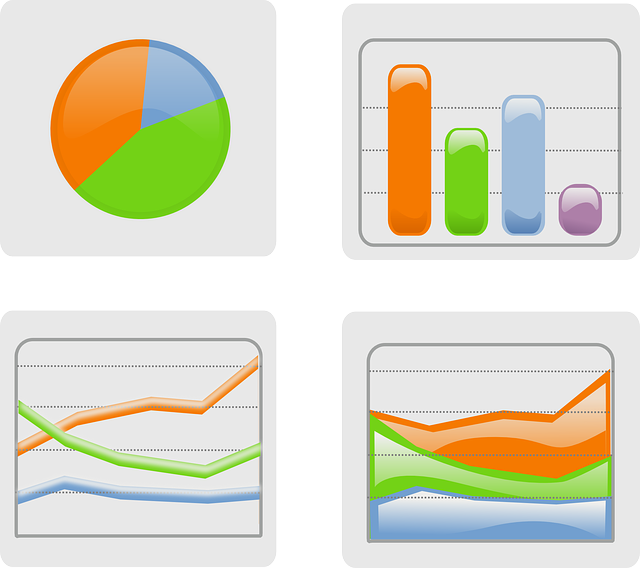# Just Graph It

Computational Thinker### Overview​

Making your own graph is a fun way to look at information! Making it online could be even better! Students can use these free sites provided to learn and practice graphing strategies that will allow them to dig deeper into information in order to gain a stronger understanding of manipulating and understanding data.

Students will:

• Be exposed to various sites that can be used for graphing data.
• Be able to explore various types of graphs that they can create.
• Be able to gather some data and create graph of their choice.

Vocabulary Words:

1. Graph: A graph is a diagram of values, usually shown as lines or bars.
2. Data: Data is facts or information that is collected together and shown as numbers.
3. Scale: A scale is the numbers that show the units used on a graph.
4. Title: A title is the label on that top that tells what the graph is showing.
5. Key: A key is a part of a map, graph, or chart that explains what the symbols mean.
6. Table: A table is a visual representation that is used to organize information and to show patterns and relationships.
7. Histogram: A histogram is a graphical display where the data is grouped into ranges (such as "40 to 49", "50 to 59", etc), and then plotted as bars. Similar to a Bar Graph, but in a Histogram each bar is for a range of data.
8. Bar Graph: A Bar graph is a graph that uses the height or length of rectangles to compare data.
9. Pictograph: A pictograph is a graph that uses pictures or symbols to show data.
10. Pie graph: A pie graph is a circular chart divided into sectors, each sector shows the relative size of each value.
11. Line plot: A line plot is a diagram showing frequency of data on a number line.
12. Vertical line (y axis): A vertical line is a line that moves up and down.
13. Horizontal line (x axis): A Horizontal line is a line that moves from side to side.

To prepare for this lesson:

See the Accommodations Page and Charts on the 21things4students website in the Teacher Resources.

Directions for this activity:

1. The teacher will say to the students, “We use graphs all the time. What do we use them for?”
• Suggested responses from the students might be; to collect data, to find trends, to compare information.
2. The teacher will then say to the students, "Today we will be looking at various tools that you can use to create graphs online."
3. The teacher will demonstrate the 3 sites or sites of their choice.
4. Go to the National Library for Virtual Manipulatives.
5. Create a quick bar graph using the graph creator.
6. Time permitting: Say, “Now you will have an opportunity to  create a graph using the data I provide on the board.”
7. Present students with some data sets and have them build a graph based on the data set given.

Different options for assessing the students:

• Observations
• Check for understanding
• Quizlet- Students can practice graphing vocabulary.
• Students can take specific data and build a graph using one of the rubrics below:

MITECS: Michigan adopted the "ISTE Standards for Students" called MITECS (Michigan Integrated Technology Competencies for Students) in 2018.

Computational Thinker
5b. Students collect data or identify relevant data sets, use digital tools to analyze them, and represent data in various ways to facilitate problem-solving and decision-making.
5c. Students break problems into component parts, extract key information, and develop descriptive models to understand complex systems or facilitate problem-solving.

Browser: Chrome, Safari, Firefox, Edge, ALL

App: